# Graph Plotting in Python | Set 1

• Difficulty Level : Easy
• Last Updated : 15 Jul, 2022

This series will introduce you to graphing in python with Matplotlib, which is arguably the most popular graphing and data visualization library for Python.
Installation
The easiest way to install matplotlib is to use pip. Type following command in terminal:

`pip install matplotlib`Getting started ( Plotting a line)

## Python

 `# importing the required module``import` `matplotlib.pyplot as plt`` ` `# x axis values``x ``=` `[``1``,``2``,``3``]``# corresponding y axis values``y ``=` `[``2``,``4``,``1``]`` ` `# plotting the points ``plt.plot(x, y)`` ` `# naming the x axis``plt.xlabel(``'x - axis'``)``# naming the y axis``plt.ylabel(``'y - axis'``)`` ` `# giving a title to my graph``plt.title(``'My first graph!'``)`` ` `# function to show the plot``plt.show()`

Output: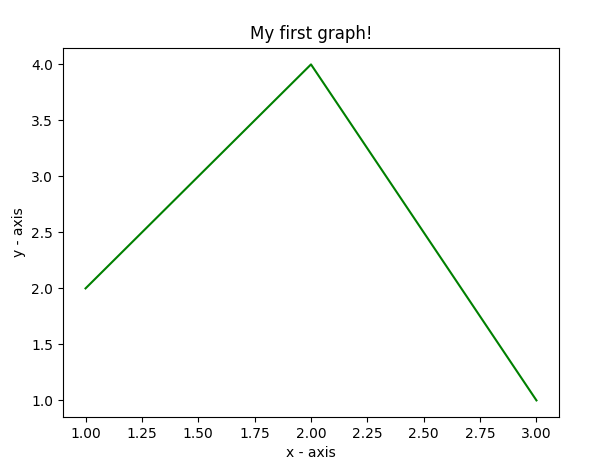The code seems self-explanatory. Following steps were followed:

• Define the x-axis and corresponding y-axis values as lists.
• Plot them on canvas using .plot() function.
• Give a name to x-axis and y-axis using .xlabel() and .ylabel() functions.
• Give a title to your plot using .title() function.
• Finally, to view your plot, we use .show() function.

Plotting two or more lines on same plot

## Python

 `import` `matplotlib.pyplot as plt`` ` `# line 1 points``x1 ``=` `[``1``,``2``,``3``]``y1 ``=` `[``2``,``4``,``1``]``# plotting the line 1 points ``plt.plot(x1, y1, label ``=` `"line 1"``)`` ` `# line 2 points``x2 ``=` `[``1``,``2``,``3``]``y2 ``=` `[``4``,``1``,``3``]``# plotting the line 2 points ``plt.plot(x2, y2, label ``=` `"line 2"``)`` ` `# naming the x axis``plt.xlabel(``'x - axis'``)``# naming the y axis``plt.ylabel(``'y - axis'``)``# giving a title to my graph``plt.title(``'Two lines on same graph!'``)`` ` `# show a legend on the plot``plt.legend()`` ` `# function to show the plot``plt.show()`

Output: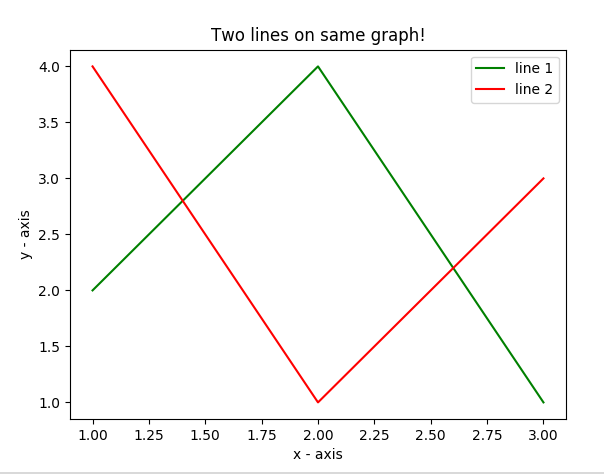• Here, we plot two lines on the same graph. We differentiate between them by giving them a name(label) which is passed as an argument of the .plot() function.
• The small rectangular box giving information about the type of line and its color is called a legend. We can add a legend to our plot using .legend() function.

Customization of Plots

Here, we discuss some elementary customizations applicable to almost any plot.

## Python

 `import` `matplotlib.pyplot as plt`` ` `# x axis values``x ``=` `[``1``,``2``,``3``,``4``,``5``,``6``]``# corresponding y axis values``y ``=` `[``2``,``4``,``1``,``5``,``2``,``6``]`` ` `# plotting the points ``plt.plot(x, y, color``=``'green'``, linestyle``=``'dashed'``, linewidth ``=` `3``,``         ``marker``=``'o'``, markerfacecolor``=``'blue'``, markersize``=``12``)`` ` `# setting x and y axis range``plt.ylim(``1``,``8``)``plt.xlim(``1``,``8``)`` ` `# naming the x axis``plt.xlabel(``'x - axis'``)``# naming the y axis``plt.ylabel(``'y - axis'``)`` ` `# giving a title to my graph``plt.title(``'Some cool customizations!'``)`` ` `# function to show the plot``plt.show()`

Output: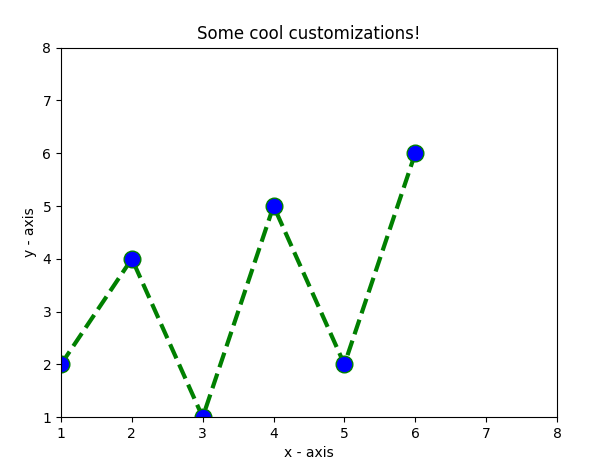As you can see, we have done several customizations like

• setting the line-width, line-style, line-color.
• setting the marker, marker’s face color, marker’s size.
• overriding the x and y-axis range. If overriding is not done, pyplot module uses the auto-scale feature to set the axis range and scale.

Bar Chart## Python

 `import` `matplotlib.pyplot as plt`` ` `# x-coordinates of left sides of bars ``left ``=` `[``1``, ``2``, ``3``, ``4``, ``5``]`` ` `# heights of bars``height ``=` `[``10``, ``24``, ``36``, ``40``, ``5``]`` ` `# labels for bars``tick_label ``=` `[``'one'``, ``'two'``, ``'three'``, ``'four'``, ``'five'``]`` ` `# plotting a bar chart``plt.bar(left, height, tick_label ``=` `tick_label,``        ``width ``=` `0.8``, color ``=` `[``'red'``, ``'green'``])`` ` `# naming the x-axis``plt.xlabel(``'x - axis'``)``# naming the y-axis``plt.ylabel(``'y - axis'``)``# plot title``plt.title(``'My bar chart!'``)`` ` `# function to show the plot``plt.show()`

Output :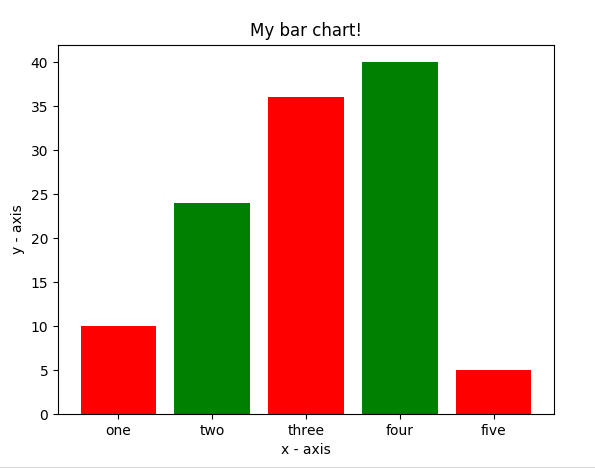• Here, we use plt.bar() function to plot a bar chart.
• x-coordinates of the left side of bars are passed along with the heights of bars.
• you can also give some names to x-axis coordinates by defining tick_labels

Histogram

## Python

 `import` `matplotlib.pyplot as plt`` ` `# frequencies``ages ``=` `[``2``,``5``,``70``,``40``,``30``,``45``,``50``,``45``,``43``,``40``,``44``,``        ``60``,``7``,``13``,``57``,``18``,``90``,``77``,``32``,``21``,``20``,``40``]`` ` `# setting the ranges and no. of intervals``range` `=` `(``0``, ``100``)``bins ``=` `10`  ` ` `# plotting a histogram``plt.hist(ages, bins, ``range``, color ``=` `'green'``,``        ``histtype ``=` `'bar'``, rwidth ``=` `0.8``)`` ` `# x-axis label``plt.xlabel(``'age'``)``# frequency label``plt.ylabel(``'No. of people'``)``# plot title``plt.title(``'My histogram'``)`` ` `# function to show the plot``plt.show()`

Output: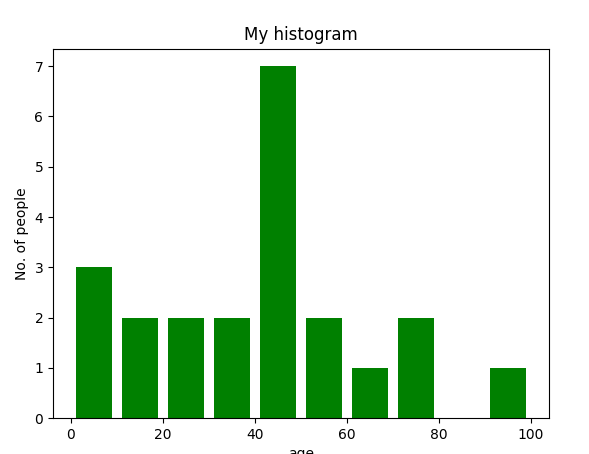• Here, we use plt.hist() function to plot a histogram.
• frequencies are passed as the ages list.
• The range could be set by defining a tuple containing min and max values.
• The next step is to “bin” the range of values—that is, divide the entire range of values into a series of intervals—and then count how many values fall into each interval. Here we have defined bins = 10. So, there are a total of 100/10 = 10 intervals.

Scatter plot

## Python

 `import` `matplotlib.pyplot as plt`` ` `# x-axis values``x ``=` `[``1``,``2``,``3``,``4``,``5``,``6``,``7``,``8``,``9``,``10``]``# y-axis values``y ``=` `[``2``,``4``,``5``,``7``,``6``,``8``,``9``,``11``,``12``,``12``]`` ` `# plotting points as a scatter plot``plt.scatter(x, y, label``=` `"stars"``, color``=` `"green"``, ``            ``marker``=` `"*"``, s``=``30``)`` ` `# x-axis label``plt.xlabel(``'x - axis'``)``# frequency label``plt.ylabel(``'y - axis'``)``# plot title``plt.title(``'My scatter plot!'``)``# showing legend``plt.legend()`` ` `# function to show the plot``plt.show()`

Output: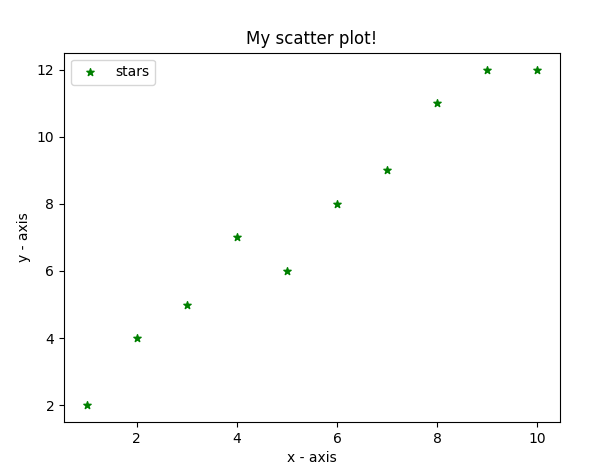• Here, we use plt.scatter() function to plot a scatter plot.
• As a line, we define x and corresponding y-axis values here as well.
• marker argument is used to set the character to use as a marker. Its size can be defined using the s parameter.

Pie-chart

## Python

 `import` `matplotlib.pyplot as plt`` ` `# defining labels``activities ``=` `[``'eat'``, ``'sleep'``, ``'work'``, ``'play'``]`` ` `# portion covered by each label``slices ``=` `[``3``, ``7``, ``8``, ``6``]`` ` `# color for each label``colors ``=` `[``'r'``, ``'y'``, ``'g'``, ``'b'``]`` ` `# plotting the pie chart``plt.pie(slices, labels ``=` `activities, colors``=``colors, ``        ``startangle``=``90``, shadow ``=` `True``, explode ``=` `(``0``, ``0``, ``0.1``, ``0``),``        ``radius ``=` `1.2``, autopct ``=` `'%1.1f%%'``)`` ` `# plotting legend``plt.legend()`` ` `# showing the plot``plt.show()`

The output of above program looks like this: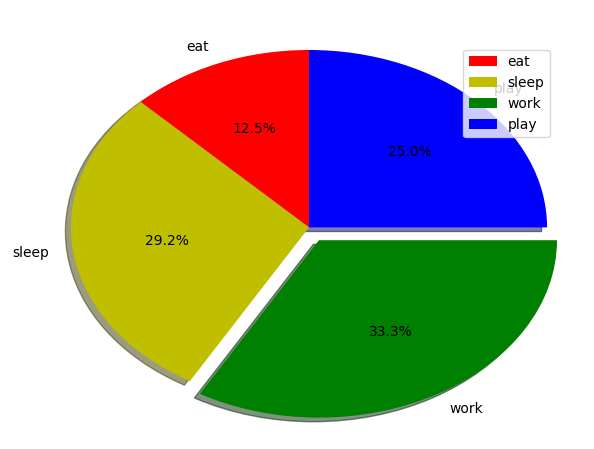• Here, we plot a pie chart by using plt.pie() method.
• First of all, we define the labels using a list called activities.
• Then, a portion of each label can be defined using another list called slices.
• Color for each label is defined using a list called colors.
• shadow = True will show a shadow beneath each label in pie chart.
• startangle rotates the start of the pie chart by given degrees counterclockwise from the x-axis.
• explode is used to set the fraction of radius with which we offset each wedge.
• autopct is used to format the value of each label. Here, we have set it to show the percentage value only upto 1 decimal place.

Plotting curves of given equation

## Python

 `# importing the required modules``import` `matplotlib.pyplot as plt``import` `numpy as np`` ` `# setting the x - coordinates``x ``=` `np.arange(``0``, ``2``*``(np.pi), ``0.1``)``# setting the corresponding y - coordinates``y ``=` `np.sin(x)`` ` `# plotting the points``plt.plot(x, y)`` ` `# function to show the plot``plt.show()`

The output

of above program looks like this: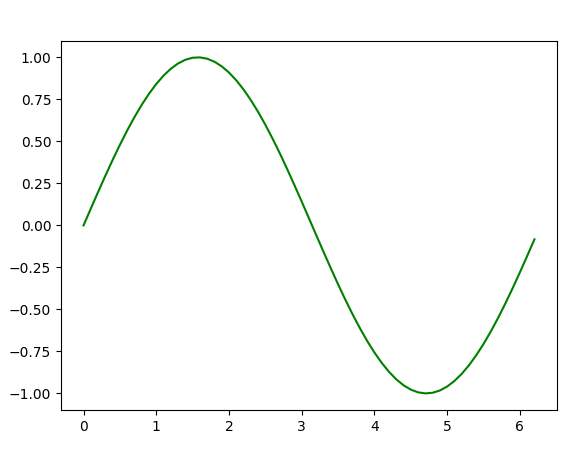Here, we use NumPy which is a general-purpose array-processing package in python.

• To set the x-axis values, we use the np.arange() method in which the first two arguments are for range and the third one for step-wise increment. The result is a NumPy array.
• To get corresponding y-axis values, we simply use the predefined np.sin() method on the NumPy array.
• Finally, we plot the points by passing x and y arrays to the plt.plot() function.

So, in this part, we discussed various types of plots we can create in matplotlib. There are more plots that haven’t been covered but the most significant ones are discussed here –

This article is contributed by Nikhil Kumar. If you like GeeksforGeeks and would like to contribute, you can also write an article using write.geeksforgeeks.org or mail your article to review-team@geeksforgeeks.org. See your article appearing on the GeeksforGeeks main page and help other Geeks.